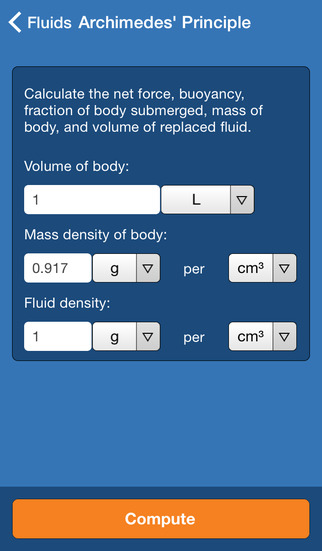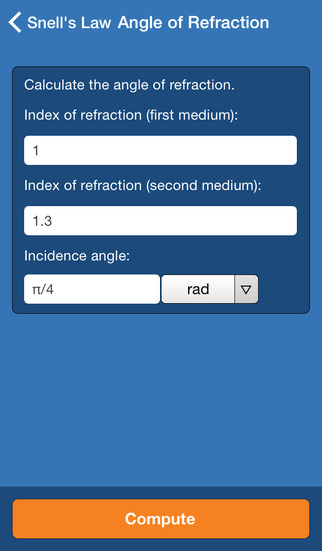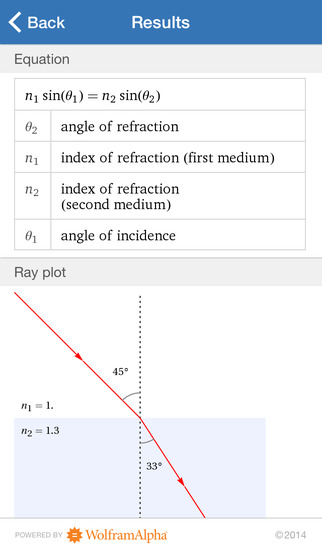0.0

# Wolfram Physics II Course Assistant

• 内容摘要
Taking introductory physics or need a quick physics reference? Then you need the Wolfram Physics II Course Assistant. This app will help you work through your homework problems, ace your tests, and learn physics concepts. Forget canned examples! The Wolfram Physics II Course Assistant solves your specific physics problems on the fly.

This app covers the following topics from Physics II:

- Calculate properties of solids, including stress, pressure, deformation, and more
- Calculate properties of fluids, including drag, buoyancy, hydrostatic pressure, and more
- Solve a variety of electricity and magnetism calculations
- Perform ray optics calculations, including Snell's law, lensmaker's equation, thin lens equation, and more
- Compute wave effects, including properties of diffraction and thin film interference
- Do common thermodynamics calculations, including the ideal gas law, Joule's first law, and more
- Look up laws of physics and common physics constants

The Wolfram Physics II Course Assistant is powered by the Wolfram|Alpha computational knowledge engine and is created by Wolfram Research, makers of Mathematica—the world's leading software system for mathematical research and education.

The Wolfram Physics II Course Assistant draws on the computational power of Wolfram|Alpha's supercomputers over a 3G, 4G, or Wi-Fi connection.

展开↓

• 版本中的新功能

展开↓

• 应用截图••#### 同类热门推荐

••AnkiMobile Flashcards 2.0.64 | 30.10MB
•••Toca Life: Hospital 1.0.1 | 220.00MB

#### 开发商的其他应用

•WolframAlpha 1.7.3 | 30.40MB
••••#### 不可错过# 安装同步助手后能更便捷的下载此应用• 免费下载游戏和应用
• 快速安装APP
• 便捷管理、优化手机
A# 连接设备至同步助手，自动安装“Wolfram Physics II Course Assistant”

### 安装步骤• 色情内容
• 赌博
• 暴力内容
• 反动内容
• 侵犯版权
• 木马病毒
• 恶意扣费
• 不良插件
• 无法安装或者启动
• 其他